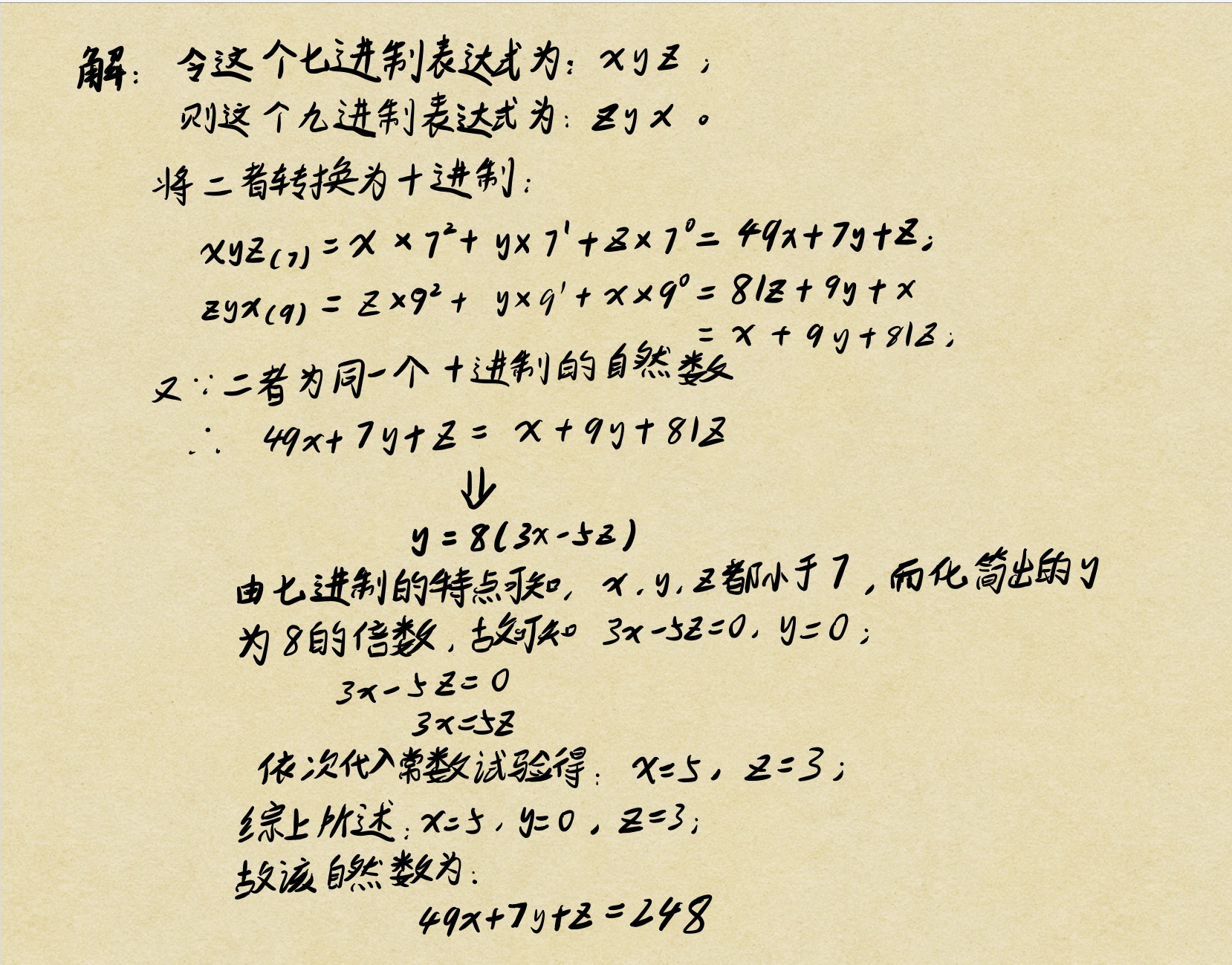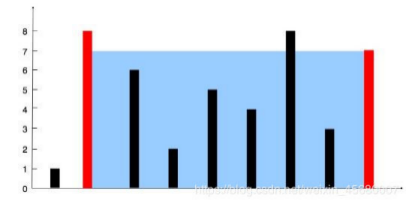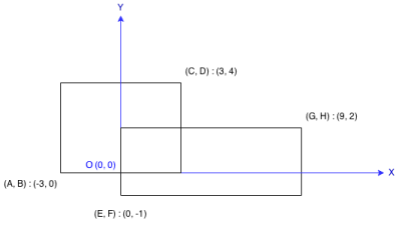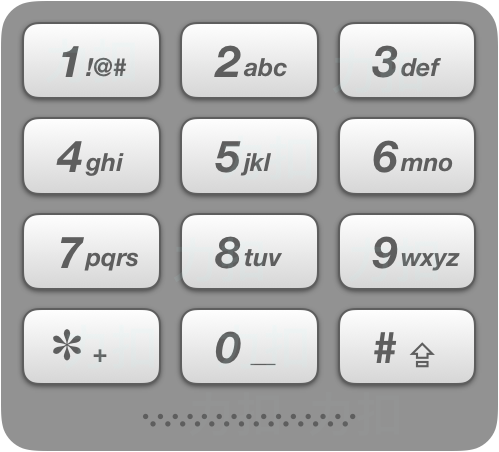• 例如123和321二者分别含有数码,且数码顺序相反。 进制:可看作是特殊进制转换为默认进制(10进制)时底数。 数学推导: 由上述数学推导过程可知,函数体内判断条件即是七进制和九进制化为十进制时二者相等,而这...
这又是一个数字问题的代码化,首先解释里面的几个概念并用数学的方法将这个三位数推导出来.
数码:数的基本单元,也就是数字.例如123和321二者分别含有三个数码,且数码顺序相反。
进制:可看作是特殊进制转换为默认进制(10进制)时的底数。
数学推导:由上述数学推导过程可知,函数体内的判断条件即是七进制和九进制化为十进制时二者相等,而这需要在所有符合七进制(七和九取并集)的三位数内进行筛选,而七进制遇七进一的特点就是突破口.
	for(i=1;i<7;i++)
for(j=0;j<7;j++)
for(k=1;k<7;k++)
if(i*9*9+j*9+k==i+j*7+k*7*7)

另外注意三位数的组成情况,因为二者数码顺序无论是正还是反都是正常的三位数,所以两端都不可以取0.
完整代码如下:
#include<stdio.h>
int main()
{
int i,j,k;
for(i=1;i<7;i++)
for(j=0;j<7;j++)
for(k=1;k<7;k++)
if(i+j*7+k*7*7==i*9*9+j*9+k)
{
printf("这个奇异的三位数是(括号内是进制):\n");
printf("%d%d%d(7)=%d%d%d(9)=%d(10)\n",k,j,i,i,j,k,i*9*9+j*9+k);
}
}

这个题对数学知识的考察要大于C语言基础内容的考察,只要进制之间的关系搞懂,那么代码化就迎刃而解了.
对我的博客或其他方面有任何见解或问题的话都可以私信我
或者联系本人QQ:3128909688
微信:DreamHerome
欢迎致电


展开全文c语言
• ## 猜数字游戏 (C#)

热门讨论 2009-09-13 09:36:56
如正确答案为5543，猜人猜5255，则在这里不能认为猜测第一个5对正确答案第二个，根据最优结果为准原理和每个数字只能有一次规则，两个比较后应该为1A1B，第一个5位子正确，记为1A；猜测数字中第三个5或...
• 4.6 网络通信的两种基本方法 36 4.7 ANSI C中提供的基本I/O函数 36 4.8 UNIX套接字API的历史 37 4.9 小结 38 进一步的研究 38 习题 38 第5章 套接字API 40 5.1 简介 40 5.2 套接字的历史 40 5.3 一协议...
• ## 你必须知道的495个C语言问题

千次下载 热门讨论 2015-05-08 11:09:25
3.18　需要根据条件把一个复杂表达式赋给两个变量中一个。可以用下面这样代码吗？((condition)?a:b)=complicated_expression; 3.19　我有些代码包含这样表达式。a?b=c:d有些编译器可以接受，有些却不能。为...C语言
• 3.18　需要根据条件把一个复杂表达式赋给两个变量中一个。可以用下面这样代码吗？((condition) ? a : b)= complicated_expression;　41　 3.19　我有些代码包含这样表达式。a ? b=c : d 有些编译器可以接受...
• 3.18　需要根据条件把一个复杂表达式赋给两个变量中一个。可以用下面这样代码吗？((condition) ? a : b)= complicated_expression;　41　 3.19　我有些代码包含这样表达式。a ? b=c : d 有些编译器可以接受...C 进阶
• /*一看到字母里面插有数字，一定要把他全部划去。 在给你纸，纸上有很多既含有字母，又含有数字句子（还包括一些标点符号）。...第一行输出字母数及其所有字母（按原来输入的相反的顺序
/*一看到字母里面插有数字，一定要把他全部划去。
在给你纸，纸上有很多既含有字母，又含有数字的句子（还包括一些标点符号）。你需要将数字找出来放在一边，并组成一个最大的数
然后逆序输出所有字母，和那个最大的数。
输入
第一行：N表示有多少组数据。
接下来的N行，每行有一句话，长度不超过1000。
输出
每句话对应输出两行。
第一行输出字母的总个数及其所有的字母（按原来输入的相反的顺序输出），中间需要一个空格分开。
第二行输出那个数字的总个数及最大的数,中间需要一个空格分开。
（如果没有字母或数字，则对应只需要输出一个0）
样例输入
2
abcabc123abc.
aaa
样例输出
9 cbacbacba
3 321
3 aaa
0*/
#include<stdio.h>
#include<string.h>
int main()
{ int t;scanf("%d",&t);while(t--){int k,b=0,i,x,j,d=0;int s2;char s,s1;scanf("%s",s);k=strlen(s);for(i=0;i<k;i++){if(s[i]>='a'&&s[i]<='z'||s[i]>='A'&&s[i]<='Z'){s1[d++]=s[i];//把字符是字母的存放在一个新的数组里}if(s[i]>='0'&&s[i]<='9'){s2[b++]=s[i];//把字符是数字的存放在一个新的数组里}}printf("%d ",d);for(i=d-1;i>=0;i--){printf("%c",s1[i]);}printf("\n"); for(i=0;i<b-1;i++){for(j=0;j<b-1-i;j++){if(s2[j]<s2[j+1]){x=s2[j];s2[j]=s2[j+1];s2[j+1]=x;}}}printf("%d ",b);for(i=0;i<b;i++){printf("%c",s2[i]);}printf("\n");}return 0;
}
展开全文• 3.12 我需要根据条件把一个复杂表达式赋值给两个变量中一 个。可以用下边这样代码吗？ ((condition) ? a : b) = complicated expression; . . . . . . . . . . . . . . . . . . . . . . . . . . . 16 目录iii ...
• // 一个包含两个元素数组 $a = "first";$a = "second"; $a[] = "third"; // 添加数组元素简单方法 // 现在$a被赋值为"third" echo count(\$a); // 打印出3，因为该是...php 入门 教程
• 电容器特点是阻碍施加在其电压变化（这一点正好和电感器相反）。结果导致电压比电流延迟90°。这关系以正弦波形式显示在图1（b）中。同时也以向量形式显示在图1（c）中。 　你还记得电感器和电容器电压...
• 　头标由四个数据元组成，它们是类CLA（ClAss）字节，命令INS（INStructic，n）字节和两个参数Pl和P2 （Parameters 1 and 2）字节，如图1所示。类字节仍旧用于识别应用和它们专有命令组。例如，GSM使用 类字节‘AO...
• 在前种格式中，会将<来源>复制至<目的地>或将多<来源>文件复制至已存在<目录>，同时设定权限模式及所有者/所属组。在第三种格式中，会创建所有指定目录及它们主目录。长选项必须用参数在使用短选项时也...
• And 运算符 执行两个表达式逻辑连接。 Array 函数 返回含一数组 变体 。 Asc 函数 返回字符串首字母 ANSI 字符代码。 赋值运算符 (=) 给变量或属性赋值。 Atn 函数 返回数反正切值。 调用语句 将控制...
• 连接运算符 (&) 强制两个表达式字符串连接。 Const 语句 声明用于字母值常数。 Cos 函数 返回角度余弦值。 CreateObject 函数 创建并返回对“自动”对象引用。 CSng 函数 返回已被转换为单精度子类型...
• ## VBSCRIPT中文手册

热门讨论 2010-11-12 10:13:06
And 运算符 执行两个表达式逻辑连接。 Array 函数 返回含一数组 变体 。 Asc 函数 返回字符串首字母 ANSI 字符代码。 赋值运算符 (=) 给变量或属性赋值。 Atn 函数 返回数反正切值。 调用语句 将控制...
• And 运算符 执行两个表达式逻辑连接。 Array 函数 返回含一数组 变体 。 Asc 函数 返回字符串首字母 ANSI 字符代码。 赋值运算符 (=) 给变量或属性赋值。 Atn 函数 返回数反正切值。 调用语句 将控制...VBScript
• And 运算符 执行两个表达式逻辑连接。 Array 函数 返回含一数组 变体 。 Asc 函数 返回字符串首字母 ANSI 字符代码。 赋值运算符 (=) 给变量或属性赋值。 Atn 函数 返回数反正切值。 调用语句 将控制...
• 快慢指针：环形数组，最多出现数组 pat型：除开自己以外乘积 ...给定一个含有正整数和负整数环形数组 nums。 如果某个索引中数 k 为正数，则向前移动 k 索引。相反，如果是负数 (-k)，则向后移动 ...
环形数组
快慢指针：最多出现两次的数组，环入口地址
pat型：除开自己的以外的乘积
Map：两数和/三数和/四数和，播放磁带，01数组，连续和为k的数组(含有负数)
滑动窗口：最长不重复子串,t步内差小于k，最小/最大的连续和为k的数组
首尾指针：水桶容量，两数和/三数和/四数和
贪心算法：合并区间，跳跃数组，矩阵面积，会议室，汽车加油
计算机数字转化
锯齿遍历，递增的三元组
环形数组：
给定一个含有正整数和负整数的环形数组 nums。 如果某个索引中的数 k 为正数，则向前移动 k 个索引。相反，如果是负数 (-k)，则向后移动 k 个索引。因为数组是环形的，所以可以假设最后一个元素的下一个元素是第一个元素，而第一个元素的前一个元素是最后一个元素。
确定 nums 中是否存在循环（或周期）。循环必须在相同的索引处开始和结束并且循环长度 > 1。此外，一个循环中的所有运动都必须沿着同一方向进行。换句话说，一个循环中不能同时包括向前的运动和向后的运动。
示例 1：
输入：[2,-1,1,2,2]
输出：true
解释：存在循环，按索引 0 -> 2 -> 3 -> 0 。循环长度为 3 。
示例 2：
输入：[-1,2]
输出：false
解释：按索引 1 -> 1 -> 1 … 的运动无法构成循环，因为循环的长度为 1 。根据定义，循环的长度必须大于 1 。
    import java.lang.*;
class Solution {
public boolean circularArrayLoop(int[] nums) {
int flag1;//作为前向或者正向运动的标志
int length=0;//长度
for(int i=0;i<nums.length;i++){
int j=i;//记录当前的位置
length=0;
if(nums[i]>0){flag1=1;}
else flag1=0;
while((nums[j]+j+nums.length)%nums.length!=i&&flag1!=-1){
if(flag1!=nums[j]/Math.abs(nums[j]))flag1=-1;
length++;
j=(nums[j]+j+nums.length)%nums.length;
}
if(length==0||flag1==-1){break;}
else{
return true;
}
}
return false;
}
}

除开自己的以外的乘积
给定长度为 n 的整数数组 nums，其中 n > 1，返回输出数组 output ，其中 output[i] 等于 nums 中除 nums[i] 之外其余各元素的乘积。
示例:
输入: [1,2,3,4]
输出: [24,12,8,6]
说明: 请不要使用除法，且在 O(n) 时间复杂度内完成此题。
答案：
除num[i]以外数字的乘积等于数字左边的乘积 乘以数字右边的乘积。转化为找"pat"类型题目
答案一：
class Solution {
public int[] productExceptSelf(int[] nums) {
int leftNums[]=new int [nums.length];//记录i数字左边的乘积
leftNums=1;//第一个数字是1
int rightNums[]=new int [nums.length];//记录i数字右边的乘积
rightNums[nums.length-1]=1;//最后一个数字1
int resultNums[]=new int [nums.length];//除了i以外数字的乘积
for(int i=1;i<nums.length;i++){
leftNums[i]=leftNums[i-1]*nums[i-1];
}
for(int i=nums.length-2;i>=0;i--){
rightNums[i]=rightNums[i+1]*nums[i+1];
resultNums[i]=leftNums[i]*rightNums[i];
}
resultNums[nums.length-1]=leftNums[nums.length-1]*rightNums[nums.length-1];
return resultNums;
}
}

答案二：
来源于leetcode解析里  LDouble 的作法
利用单数组res,k记录
class Solution {
public int[] productExceptSelf(int[] nums) {
int[] res = new int[nums.length];
int k = 1;
for(int i = 0; i < res.length; i++){
res[i] = k;
k = k * nums[i]; // 此时数组存储的是除去当前元素左边的元素乘积
}
k = 1;
for(int i = res.length - 1; i >= 0; i--){
res[i] *= k; // k为该数右边的乘积。
k *= nums[i]; // 此时数组等于左边的 * 该数右边的。
}
return res;
}
}

两数和：
解析：利用辅助数组(或者Map)
两次遍历:
 class Solution {
public int[] twoSum(int[] nums, int target) {
Map<Integer,Integer> map =new HashMap<>();
for(int i=0;i<nums.length;i++){
map.put(target-nums[i],i);
}
for(int i=0;i<nums.length;i++){
if(map.containsKey(nums[i])&&map.get(nums[i])!=i){
return new int[]{i,map.get(nums[i])};
}
}
return null;
}
}

一次遍历：
 class Solution {
public int[] twoSum(int[] nums, int target) {
Map<Integer,Integer> map =new HashMap<>();
for(int i=0;i<nums.length;i++){
if(map.containsKey(target-nums[i])){
return new int []{map.get(target-nums[i]),i};
}
map.put(nums[i],i);
}
return null;
}
}

三数和：
给定一个包含 n 个整数的数组 nums，判断 nums 中是否存在三个元素 a，b，c ，使得 a + b + c = 0 ？找出所有满足条件且不重复的三元组。
注意：答案中不可以包含重复的三元组。
例如, 给定数组 nums = [-1, 0, 1, 2, -1, -4]，
满足要求的三元组集合为：
[
[-1, 0, 1],
[-1, -1, 2]
]
import java.lang.*;
import java.util.*;

class Solution {
public List<List<Integer>> threeSum(int[] nums) {
List<List<Integer>> list=new LinkedList<>();
int low,high;
int len=nums.length;
Arrays.sort(nums);
for(int i=0;i<len-2;i++){
if(i>0&&nums[i]==nums[i-1]) continue;
low=i+1;
high=len-1;
while(low<high){
int sum=nums[i]+nums[low]+nums[high];
if(sum==0){
list.add(Arrays.asList(nums[i],nums[low],nums[high]));
low++;high--;
while(low<high&&nums[low-1]==nums[low])low++;
while(low<high&&nums[high+1]==nums[high])high--;
}
else if(sum<0)low++;
else high--;
}
}
return list;
}
}

题目四：四数和
给定一个包含 n 个整数的数组 nums 和一个目标值 target，判断 nums 中是否存在四个元素 a，b，c 和 d ，使得 a + b + c + d 的值与 target 相等？找出所有满足条件且不重复的四元组。
注意：
答案中不可以包含重复的四元组。
示例：
给定数组 nums = [1, 0, -1, 0, -2, 2]，和 target = 0。
满足要求的四元组集合为：
[
[-1,  0, 0, 1],
[-2, -1, 1, 2],
[-2,  0, 0, 2]
]
import java.lang.*;
import java.util.*;

class Solution {
public List<List<Integer>> fourSum(int[] nums, int target) {
List<List<Integer>> list=new LinkedList<>();
int len=nums.length;
int low,high;
Arrays.sort(nums);
for(int i=0;i<len-3;i++){
if(i>0&&nums[i]==nums[i-1]) continue;
for(int j=i+1;j<len-2;j++){
if(j>i+1&&nums[j]==nums[j-1]) continue;
low=j+1;high=len-1;

while(low<high){
int sum=nums[i]+nums[j]+nums[low]+nums[high];
if(sum==target){
list.add(Arrays.asList(nums[i],nums[j],nums[low],nums[high]));
low++;high--;
while(low<high&&nums[low]==nums[low-1])low++;
while(low<high&&nums[high]==nums[high+1])high--;
}
else if(sum<target)low++;
else high--;
}
}
}
return list;
}
}

最接近的三数和
给定一个包括 n 个整数的数组 nums 和 一个目标值 target。找出 nums 中的三个整数，使得它们的和与 target 最接近。返回这三个数的和。假定每组输入只存在唯一答案。
例如，给定数组 nums = [-1，2，1，-4], 和 target = 1.
与 target 最接近的三个数的和为 2. (-1 + 2 + 1 = 2).
class Solution {
public int threeSumClosest(int[] nums, int target) {
Arrays.sort(nums);
int ans=nums+nums+nums;
for(int i=0;i<nums.length-2;i++){
int begin=i+1,end=nums.length-1;
while(begin<end){
int value=nums[i]+nums[begin]+nums[end];
if(Math.abs(target-value)<Math.abs(target-ans)){
ans=value;
}
if(value>target)
end--;
else if(value < target)
begin++;
else return ans;
}
}
return ans;
}
}

01连续子数组
给定一个二进制数组, 找到含有相同数量的 0 和 1 的最长连续子数组（的长度）。
示例 1:
输入: [0,1]
输出: 2
说明: [0, 1] 是具有相同数量0和1的最长连续子数组。
示例 2:
输入: [0,1,0]
输出: 2
说明: [0, 1] (或 [1, 0]) 是具有相同数量0和1的最长连续子数组。
首先对原数组做处理，将所有的0都变为-1；这样一来 “含有相同数量的 0 和 1 的连续数组” 就等价为 “元素值总和为0的连续数组”。
其后，从头扫一遍数组，并记录当前的前缀和的值，将该值和对应的下标存入到一个标记数组或哈希表中。若该前缀和的值已出现过（即标记数组或哈希中已存在），则说明标记中的下标到当前扫描的下标的这段数组的总和值是为0的。
打个例子： [ -1，-1，-1，1，-1，1，-1，1，-1，-1，-1，-1 ] 在扫描完到第四个元素时，前缀和为-2 且未记录过，则将值-2和下标3记录起来。当扫描到  [ -1，-1，-1，1，-1，-1，1，1，-1，-1，-1，-1 ]  ， 此时得到的前缀和为-2，且知道标记中有记录过-2，则说明此刻下标到之前记录的下标的这段数组总和为0  [ -1，-1，-1，1，-1，-1，1，1，-1，-1，-1，-1 ]
import java.lang.*;
class Solution {
public int findMaxLength(int[] nums) {
int len=nums.length;
int arr[]=new int [2*len+1];
Arrays.fill(arr, -2);//辨别此位置之前没有数字
int count=0,maxlen=0;
arr[len]=-1;//数组开头处count=0，位置为-1
for(int i=0;i<len;i++){
count=count+(nums[i]==1?1:-1);
if(arr[count+len]>=-1){//即之前出现过此值,说明两个值序列间01数量相同
maxlen=Math.max(maxlen,i-arr[count+len]);
}
else arr[count+len]=i;//只用保留第一次出现此值得位置，后面的不用保留
}
return maxlen;
}
}

含有重复字符的 最长子串：
给定一个字符串，请你找出其中不含有重复字符的 最长子串 的长度。
示例 1
输入: “abcabcbb”
输出: 3
解释: 因为无重复字符的最长子串是 “abc”，所以其长度为 3。
示例 2:
输入: “bbbbb”
输出: 1
解释: 因为无重复字符的最长子串是 “b”，所以其长度为 1。
示例 3:
输入: “pwwkew”
输出: 3
解释: 因为无重复字符的最长子串是 “wke”，所以其长度为 3。
请注意，你的答案必须是 子串 的长度，“pwke” 是一个子序列，不是子串。
解析：利用滑动窗口。一个队列,比如例题中的 abcabcbb，进入这个队列（窗口）为 abc 满足题目要求。当再进入 a，队列变成了 abca，这时候不满足要求。我们需要对窗口进行移动，即判断
left = Math.max(left,map.get(s.charAt(i)) + 1);
class Solution {
public int lengthOfLongestSubstring(String s) {
if (s.length()==0) return 0;
HashMap<Character, Integer> map = new HashMap<Character, Integer>();
int max = 0;
int left = 0;
for(int i = 0; i < s.length(); i ++){
if(map.containsKey(s.charAt(i))){
left = Math.max(left,map.get(s.charAt(i)) + 1);
}
map.put(s.charAt(i),i);
max = Math.max(max,i-left+1);
}
return max;
}
}

播放磁带：：
在歌曲列表中，第 i 首歌曲的持续时间为 time[i] 秒。
返回其总持续时间（以秒为单位）可被 60 整除的歌曲对的数量。形式上，我们希望索引的数字  i < j 且有 (time[i] + time[j]) % 60 == 0。
示例 1：
输入：[30,20,150,100,40]
输出：3
解释：这三对的总持续时间可被 60 整数：
(time = 30, time = 150): 总持续时间 180
(time = 20, time = 100): 总持续时间 120
(time = 20, time = 40): 总持续时间 60
示例 2：
输入：[60,60,60]
输出：3
解释：所有三对的总持续时间都是 120，可以被 60 整数。
答案一：
class Solution {
public int numPairsDivisibleBy60(int[] time) {
Map<Integer,Integer> map=new HashMap<>();
int count=0;
int len=time.length;//单独运算，防止for循环中每一次遍历都调用此方法，节省时间
for(int i=0;i<len;i++){
time[i]=time[i]%60;
int needTime=(60-time[i])%60;
//判断对于needTime是否存在于之相补为60的数字
Integer value1=map.get(needTime);
if(value1!=null){
count+=value1;
}
//将此数字将入map
Integer value2=map.get(time[i]);
if(value2==null)map.put(time[i],1);
else map.put(time[i],value2+1);
}
return count;
}
}

注意：
1：相当于依顺序遍历数组，对于先加入的数字，即使存在满足情况但是未能匹配，后加入的数字也会对其进行匹配
2： Map<Integer,Integer> map=new HashMap<>();泛型map，需要new HashMap<>()而不能new Map<>()对其初始化。同时，put(),get()的值也是包装类例如Integer,只不过现在ja
水桶水量：
给定 n 个非负整数 a1，a2，…，an，每个数代表坐标中的一个点 (i, ai) 。在坐标内画 n 条垂直线，垂直线 i 的两个端点分别为 (i, ai) 和 (i, 0)。找出其中的两条线，使得它们与 x 轴共同构成的容器可以容纳最多的水。
说明：你不能倾斜容器，且 n 的值至少为 2。图中垂直线代表输入数组 [1,8,6,2,5,4,8,3,7]。在此情况下，容器能够容纳水（表示为蓝色部分）的最大值为 49。
示例:
输入: [1,8,6,2,5,4,8,3,7]
输出: 49
解析：两端指针，移动时会将较小的边指针向里移动一位（因为若移动较大的边的指针，面积一定减少）
class Solution {
public int maxArea(int[] height) {
int max=0;
int len=height.length;
int i=0,j=len-1;
while(i!=j){
max=Math.max(Math.min(height[i],height[j])*(j-i),max);
if(height[i]>height[j])j--;
else i++;
}
return max;
}
}

跳跃数组：
给定一个非负整数数组，你最初位于数组的第一个位置。
数组中的每个元素代表你在该位置可以跳跃的最大长度。
判断你是否能够到达最后一个位置。
示例 1:
输入: [2,3,1,1,4]
输出: true
解释: 从位置 0 到 1 跳 1 步, 然后跳 3 步到达最后一个位置。
示例 2:
输入: [3,2,1,0,4]
输出: false
解释: 无论怎样，你总会到达索引为 3 的位置。但该位置的最大跳跃长度是 0 ， 所以你永远不可能到达最后一个位置。
解法一：
class Solution {
public boolean canJump(int[] nums) {
if(nums.length==0||nums.length==1)return true;
int nowValue=0;
int farValue=nums[nowValue];
int len=nums.length;
while(nowValue!=farValue&&farValue<len-1){
int value=farValue;//需要存储前一次farValue的值
for(int i=nowValue;i<=farValue;i++){
if(i>=len-1)return true;
farValue=Math.max(farValue,i+nums[i]);
}
nowValue=farValue;
}
if(farValue>=len-1)return true;
return false;
}
}

重叠区间：
给出一个区间的集合，请合并所有重叠的区间。
示例 1:
输入: [[1,3],[2,6],[8,10],[15,18]]
输出: [[1,6],[8,10],[15,18]]
解释: 区间 [1,3] 和 [2,6] 重叠, 将它们合并为 [1,6].
示例 2:
输入: [[1,4],[4,5]]
输出: [[1,5]]
解释: 区间 [1,4] 和 [4,5] 可被视为重叠区间。
解析：先将区间按左边界排序。之后只需要判断【区间i的右边界，区间i+1的左边界】的关系即可
class Solution {
public int[][] merge(int[][] intervals) {
List<int[]> result=new LinkedList<>();
if(intervals.length==0||intervals.length==0)return result.toArray(new int []);
Arrays.sort(intervals,(a,b)->a-b);
int i=0,len=intervals.length;
while(i<len){
int left=intervals[i];
int right=intervals[i];
while(i+1<len&&right>=intervals[i+1]){
right=Math.max(right,intervals[i+1]);i++;
}
result.add(new int[]{left, right});;i++;
}
return result.toArray(new int []);
}
}

t步内差小于k
给定一个整数数组，判断数组中是否有两个不同的索引 i 和 j，使得 nums [i] 和 nums [j] 的差的绝对值最大为 t，并且 i 和 j 之间的差的绝对值最大为 ķ。
示例 1:
输入: nums = [1,2,3,1], k = 3, t = 0
输出: true
示例 2:
输入: nums = [1,0,1,1], k = 1, t = 2
输出: true
示例 3:
输入: nums = [1,5,9,1,5,9], k = 2, t = 3
输出: false
解法一：利用滑动窗口 大小为k
class Solution {
public boolean containsNearbyAlmostDuplicate(int[] nums, int k, int t) {
List<Integer> list=new ArrayList<>();
for(int i=0;i<nums.length;i++){
for (int j= 0; j<list.size(); j++) {
if(Math.abs((long)list.get(j)-(long)nums[i])<=t)return true;
}
list.add(nums[i]);
if(list.size()>k)list.remove(0);
}
return false;
}
}

矩阵面积：
leetcode223
在二维平面上计算出两个由直线构成的矩形重叠后形成的总面积。
每个矩形由其左下顶点和右上顶点坐标表示，如图所示。示例:
输入: -3, 0, 3, 4, 0, -1, 9, 2
输出: 45
解析：
对于相离情况，将矩阵A不动，其周围空间化作四等分【左右上下】
对于相交情况，关键在于找相交矩阵，相交矩阵的四条边都来自于两个矩阵的四条边
class Solution {
public int computeArea(int A, int B, int C, int D, int E, int F, int G, int H) {
//相离的情况
int value=(C-A)*(D-B)+(G-E)*(H-F);
if(F>D||H<B||C<E||G<A)return value ;
int a,b,c,d;//重叠矩形的四条边
a=Math.max(A,E);
b=Math.max(B,F);
c=Math.min(C,G);
d=Math.min(D,H);
int result=Math.abs(a-c)*Math.abs(b-d);
return value-result;
}
}

计算机数字转化：
给定一个仅包含数字 2-9 的字符串，返回所有它能表示的字母组合。
给出数字到字母的映射如下（与电话按键相同）。注意 1 不对应任何字母。
示例:
输入：“23”
输出：[“ad”, “ae”, “af”, “bd”, “be”, “bf”, “cd”, “ce”, “cf”].
说明:
解析：利用队列的思想，先进先出进行组合
new String[] {“0”, “1”, “abc”, “def”, “ghi”, “jkl”, “mno”, “pqrs”, “tuv”, “wxyz”};这样设计的目的是下表与字符串相对应，如2-,"abc"leetcode17
给定一个仅包含数字 2-9 的字符串，返回所有它能表示的字母组合。
给出数字到字母的映射如下（与电话按键相同）。注意 1 不对应任何字母。示例:
输入：“23”
输出：[“ad”, “ae”, “af”, “bd”, “be”, “bf”, “cd”, “ce”, “cf”].
说明:
解析：利用队列的思想，先进先出进行组合
new String[] {“0”, “1”, “abc”, “def”, “ghi”, “jkl”, “mno”, “pqrs”, “tuv”, “wxyz”};这样设计的目的是下表与字符串相对应，如2-,“abc”
public List<String> letterCombinations(String digits) {
LinkedList<String> ans = new LinkedList<String>();
if(digits.isEmpty()) return ans;
String[] mapping = new String[] {"0", "1", "abc", "def", "ghi", "jkl", "mno", "pqrs", "tuv", "wxyz"};
ans.add("");
for(int i =0; i<digits.length();i++){
int x = Character.getNumericValue(digits.charAt(i));
while(ans.peek().length()==i){
String t = ans.remove();
for(char s : mapping[x].toCharArray())
ans.add(t+s);
}
}
return ans;
}

public List<String> letterCombinations(String digits) {
LinkedList<String> ans = new LinkedList<String>();
if(digits.isEmpty()) return ans;
String[] mapping = new String[] {"0", "1", "abc", "def", "ghi", "jkl", "mno", "pqrs", "tuv", "wxyz"};
ans.add("");
for(int i =0; i<digits.length();i++){
int x = Character.getNumericValue(digits.charAt(i));
while(ans.peek().length()==i){
String t = ans.remove();
for(char s : mapping[x].toCharArray())
ans.add(t+s);
}
}
return ans;
}

汽车加油：
在一条环路上有 N 个加油站，其中第 i 个加油站有汽油 gas[i] 升。
你有一辆油箱容量无限的的汽车，从第 i 个加油站开往第 i+1 个加油站需要消耗汽油 cost[i] 升。你从其中的一个加油站出发，开始时油箱为空。
如果你可以绕环路行驶一周，则返回出发时加油站的编号，否则返回 -1。
说明:
如果题目有解，该答案即为唯一答案。
输入数组均为非空数组，且长度相同。
输入数组中的元素均为非负数。
示例 1:
输入:
gas  = [1,2,3,4,5]
cost = [3,4,5,1,2]
输出: 3
解释:
从 3 号加油站(索引为 3 处)出发，可获得 4 升汽油。此时油箱有 = 0 + 4 = 4 升汽油
开往 4 号加油站，此时油箱有 4 - 1 + 5 = 8 升汽油
开往 0 号加油站，此时油箱有 8 - 2 + 1 = 7 升汽油
开往 1 号加油站，此时油箱有 7 - 3 + 2 = 6 升汽油
开往 2 号加油站，此时油箱有 6 - 4 + 3 = 5 升汽油
开往 3 号加油站，你需要消耗 5 升汽油，正好足够你返回到 3 号加油站。
因此，3 可为起始索引。
示例 2:
输入:
gas  = [2,3,4]
cost = [3,4,3]
输出: -1
解释:
你不能从 0 号或 1 号加油站出发，因为没有足够的汽油可以让你行驶到下一个加油站。
我们从 2 号加油站出发，可以获得 4 升汽油。 此时油箱有 = 0 + 4 = 4 升汽油
开往 0 号加油站，此时油箱有 4 - 3 + 2 = 3 升汽油
开往 1 号加油站，此时油箱有 3 - 3 + 3 = 3 升汽油
你无法返回 2 号加油站，因为返程需要消耗 4 升汽油，但是你的油箱只有 3 升汽油。
因此，无论怎样，你都不可能绕环路行驶一周。
解析：想清楚两点
1：如果0-i不能达到，则直接从i+1开始(0-i之间任何节点都不能到达)
2：如果总的gas>总的cost，则一定存在某节点能遍历。则从遍历到n-1的那个i+1开始的结点即是所求得节点
class Solution {
public int canCompleteCircuit(int[] gas, int[] cost) {
int total = 0, sum = 0, start = 0;
for (int i = 0; i < gas.length; ++i) {
total += gas[i] - cost[i];
sum += gas[i] - cost[i];
if (sum < 0) {
start = i + 1;
sum = 0;
}
}
return (total < 0) ? -1 : start; // total 小于 0，说明总消耗大于总油量，那必定无法行驶完所有站点
}
}

环入口地址
解析：
1：假设链表环外长度F，环内长度C
2：slow节点走了长度F，fast节点走了2F，此时在环内的h=Fmod C点
3：再走C-h步两者相遇，slow=(C-h)mod C  fast=(2C-2h+h)mod C=(2C-h)modC=(C-h)mod C
4：此时新设指针prt，让fast和pr同时前进，走F步两者在入口处相遇。fasto=(C-FmodC+F)modC=0
public class Solution {
private ListNode getIntersect(ListNode head) {
ListNode tortoise = head;
ListNode hare = head;
while (hare != null && hare.next != null) {
tortoise = tortoise.next;
hare = hare.next.next;
if (tortoise == hare) {
return tortoise;
}
}
return null;
}

public ListNode detectCycle(ListNode head) {
if (head == null) {
return null;
}
ListNode intersect = getIntersect(head);
if (intersect == null) {
return null;
}
ListNode ptr1 = head;
ListNode ptr2 = intersect;
while (ptr1 != ptr2) {
ptr1 = ptr1.next;
ptr2 = ptr2.next;
}
return ptr1;
}
}

长度为sum的最短连续子数组
给定一个含有 n 个正整数的数组和一个正整数 s ，找出该数组中满足其和 ≥ s 的长度最小的连续子数组。如果不存在符合条件的连续子数组，返回 0。
示例:
输入: s = 7, nums = [2,3,1,2,4,3]
输出: 2
解释: 子数组 [4,3] 是该条件下的长度最小的连续子数组。
解析：要有意识，遇见连续数组，运用滑动窗口
class Solution {
public int minSubArrayLen(int s, int[] nums) {
if(nums.length==0)return 0;
int begin=0,end=0,minLength=Integer.MAX_VALUE,sum=nums[begin];
while(begin<=end&&end<nums.length){
if(sum>s){
minLength=Math.min(minLength,end-begin+1);
sum=sum-nums[begin];
begin++;
}
if(sum<s){
if(end==nums.length-1)break;
end++;
sum=sum+nums[end];
}
if(sum==s){
minLength=Math.min(minLength,end-begin+1);
begin++;end++;
if(end==nums.length)break;
sum=sum-nums[begin-1]+nums[end];
}
}
return minLength==Integer.MAX_VALUE?0:minLength;
}
}

会议室：
给定一个会议时间安排的数组，每个会议时间都会包括开始和结束的时间 [[s1,e1],[s2,e2],…] (si < ei)，为避免会议冲突，同时要考虑充分利用会议室资源，请你计算至少需要多少间会议室，才能满足这些会议安排。
示例 1:
输入: [[0, 30],[5, 10],[15, 20]]
输出: 2
示例 2:
输入: [[7,10],[2,4]]
输出: 1
class Solution implements Comparator<int []> {
public int minMeetingRooms(int[][] intervals) {
if(intervals.length==0)return 0;
int []endTime =new int[intervals.length];
int count=0;
Arrays.sort(intervals,this);
endTime[count]=intervals;
int length=1,flag=0;
while(length<intervals.length){
for(int i=0;i<=count;i++){
if(intervals[length]>=endTime[i]){
endTime[i]=intervals[length];
flag=1;
break;
}
}
if(flag==0){
count++;
endTime[count]=intervals[length];
}
length++;
flag=0;
}
return count+1;
}
public int compare(int a[],int b[]){
return a-b;
}
}

小于target的三数和
给定一个长度为 n 的整数数组和一个目标值 target，寻找能够使条件 nums[i] + nums[j] + nums[k] < target 成立的三元组  i, j, k 个数（0 <= i < j < k < n）。
示例：
输入: nums = [-2,0,1,3], target = 2
输出: 2
解释: 因为一共有两个三元组满足累加和小于 2:
[-2,0,1]
[-2,0,3]
	class Solution {
public int threeSumSmaller(int[] nums, int target) {
if(nums.length<3)return 0;
Arrays.sort(nums);
int result=0;
for(int i=0;i<nums.length-2;i++){
int left=i+1,right=nums.length-1;
while(left<right){
if(nums[left]+nums[right]>=target-nums[i])right--;
else{
result+=right-left;
left++;right=nums.length-1;
}
}
}
return result;
}
}

锯齿遍历：
给出两个一维的向量，请你实现一个迭代器，交替返回它们中间的元素。
示例:
输入:
v1 = [1,2]
v2 = [3,4,5,6]
输出: [1,3,2,4,5,6]
解析: 通过连续调用 next 函数直到 hasNext 函数返回 false，
next 函数返回值的次序应依次为: [1,3,2,4,5,6]。
拓展：假如给你 k 个一维向量呢？你的代码在这种情况下的扩展性又会如何呢?
拓展声明：
“锯齿” 顺序对于 k > 2 的情况定义可能会有些歧义。所以，假如你觉得 “锯齿” 这个表述不妥，也可以认为这是一种 “循环”。例如：
输入:
[1,2,3]
[4,5,6,7]
[8,9]
输出: [1,4,8,2,5,9,3,6,7].
public class ZigzagIterator {
Queue<Iterator<Integer>> queue = new LinkedList<>();
public ZigzagIterator(List<Integer> v1, List<Integer> v2) {
Iterator<Integer> it1 = v1.iterator();
Iterator<Integer> it2 = v2.iterator();
if (it1.hasNext()) {
queue.add(it1);
}
if (it2.hasNext()) {
queue.add(it2);
}
}

public int next() {
Iterator<Integer> it = queue.poll();
int v = it.next();
if (it.hasNext()) {
queue.add(it);
}
return v;
}
public boolean hasNext() {
if (!queue.isEmpty()) {
return true;
}
return false;
}
}

最大连续和为k的数组（含有负数）
给定一个数组 nums 和一个目标值 k，找到和等于 k 的最长子数组长度。如果不存在任意一个符合要求的子数组，则返回 0。
注意:
nums 数组的总和是一定在 32 位有符号整数范围之内的。
示例 1:
输入: nums = [1, -1, 5, -2, 3], k = 3
输出: 4
解释: 子数组 [1, -1, 5, -2] 和等于 3，且长度最长。
示例 2:
输入: nums = [-2, -1, 2, 1], k = 1
输出: 2
解释: 子数组 [-1, 2] 和等于 1，且长度最长。
class Solution {
public int maxSubArrayLen(int[] nums, int k) {
if(nums == null || nums.length == 0){
return 0;
}
HashMap<Integer, Integer> map = new HashMap<>();
int sum = 0;
int res = 0;
for(int i=0; i<nums.length; i++){
sum += nums[i];
if(sum == k){
res = i+1;
}else if(map.containsKey(sum-k)){
res = Math.max(res, i+1-(map.get(sum-k)+1));
}
if(!map.containsKey(sum)){
map.put(sum,i);
}
}
return res;
}
}

递增的三元组
给定一个未排序的数组，判断这个数组中是否存在长度为 3 的递增子序列。
数学表达式如下:
如果存在这样的 i, j, k,  且满足 0 ≤ i < j < k ≤ n-1，
使得 arr[i] < arr[j] < arr[k] ，返回 true ; 否则返回 false 。
说明: 要求算法的时间复杂度为 O(n)，空间复杂度为 O(1) 。
示例 1:
输入: [1,2,3,4,5]
输出: true
示例 2:
输入: [5,4,3,2,1]
输出: false
class Solution {
public boolean increasingTriplet(int[] nums) {
int index1=Integer.MAX_VALUE,index2=Integer.MAX_VALUE;
for(int i=0;i<nums.length;i++){
if(nums[i]<index1){
index1=nums[i];
}
else if(nums[i]>index1&&nums[i]<index2){
index2=nums[i];
}
else if(nums[i]>index2)return true;
}
return false;
}
}



展开全文• 你可以看见如果你没有用我们提到的两个字符（最后一个例子），就是说 模式（正则表达式） 可以出现在被检验字符串的任何地方，你没有把他锁定到两边 这里还有几个字符 '*', '+',和 '?', 他们用来表示一个字符可以...Java
• 但是,它要求开始和结束引号匹配(例如两个都是双引号或者都是单引号): /[' "] [^ ' "]*[' "]/ 如果要求开始和结束引号匹配,我们可以使用如下引用: /( [' "] ) [^ ' "] * \1/ \1匹配是第一个代括号...正则表达式
• o 4.12 我需要根据条件把一个复杂表达式赋值给两个变量中一个。可以用下边这样代码吗？ ((condition) ? a : b) = complicated_expression; * 5. 指针 o 5.1 我想声明一个指针并为它分配一些空间, 但却...
• （但是请注意：两个不同但结构上等效委托类型实例可能会比较为相等）,准确地说，两个具有相同参数列表、签名和返回类型不同委托类型被认为是不同委托类型。委托实例所封装方法集合称为调用列表。 5, ...C#
• 26．设一组初始记录关键字序列为(25，50，15，35，80，85，20，40，36，70)，其中含有5长度为2有序子表，则用归并排序方法对该记录关键字序列进行一趟归并后结果为（ ）。 (A) 15，25，35，50，20，40，80，...数据结构题